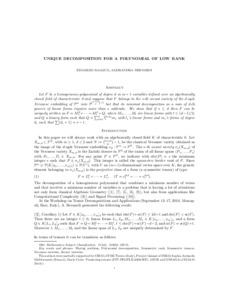# Unique decomposition for a polynomial of low rank

Ballico, Edoardo ; Bernardi, Alessandra (2011) Unique decomposition for a polynomial of low rank. [Preprint]
Full text available as:Preview PDF Download (228kB) | Preview

## Abstract

Let $F$ be a homogeneous polynomial of degree $d$ in $m+1$ variables defined over an algebraically closed field of characteristic 0 and suppose that $F$ belongs to the $s$-th secant variety of the $d$-uple Veronese embedding of $\mathbb{P}^m$ into $\mathbb{P}^{{m+d\choose d}-1}$ but that its minimal decomposition as a sum of $d$-th powers of linear forms requires more than $s$ addenda. We show that if $s\leq d$ then $F$ can be uniquely written as $F=M_1^d+\cdots + M_t^d+Q$, where $M_1, \ldots , M_t$ are linear forms with $t\leq (d-1)/2$, and $Q$ a binary form such that $Q=\sum_{i=1}^q l_i^{d-d_i}m_i$ with $l_i$'s linear forms and $m_i$'s forms of degree $d_i$ such that $\sum (d_i+1)=s-t$.

Abstract
Document type
Preprint
Creators
CreatorsAffiliationORCID
Ballico, Edoardo
Bernardi, Alessandra
Subjects
DOI
Deposit date
02 Aug 2011 16:26